Latest Banking jobs   »

# Reasoning Quizzes Quiz For IRDA AM 2023- 18th April

Directions (1-3): Study the following information carefully and answer the questions given below:
There are six members in a family of three generation i.e. A, B, C, D, E, F. There two are married couples in the family. D is son in law of B.A is grandmother of F. E is son of C. E and F have opposite gender.

Q1. Who among the following person is grandfather of E?
(a)C
(b)A
(c)B
(d)D
(e) None of these

Q2. How is D related to A?
(a)Father
(b)Daughter
(c)Son
(d)Son in law
(e) None of these

Q3. How is F related to C?
(a) Mother-in-law
(b)Daughter
(c)Mother
(d)Son
(e) None of these

Direction (4-6): Study the given information and answer the following questions.
There are eight members in a family of three generation having two married couples. G and H are siblings. E is brother-in-law of F. E is the only son of A. F has only two children. B is married to A. A has no siblings. D is aunt of E. F has no siblings and married to C. G is the only son of C.

Q4. If B is a female member then how A is related to F?
(a) Father-in-law
(b) Brother
(c) Father
(d) None of these
(e) Brother-in-law

Q5. How many female members are there in a family?
(a) Three
(b) Four
(c) None of these
(d) Five
(e) Can’t be determined

Q6. How E is related to G?
(a)Father-in-law
(b)Paternal uncle
(c)Maternal uncle
(d)None of these
(e)Brother-in-law

Direction (7-9): Study the following information carefully and answer the questions given below:
There are eight members in a family of three generations. G is the father-in-law of C. C is the mother of F. F and D are siblings. D is the only nephew of E. E is unmarried. B is the only son of A. E is not the sibling of C. A is the grandmother of F.

Q7. How is E related to F?
(a)Paternal uncle
(b)Maternal uncle
(c)Paternal aunt
(d)Maternal Aunt
(e)None of these

Q8. How many male members are there in the family?
(a)Three
(b)Four
(c)Five
(d)Six
(e)Two

Q9. If E is married to J, then how is G related to J?
(a)Father
(b)Father-in-law
(c)Brother
(d)Son-in-law
(e)None of these

Direction (10-12): Study the following information carefully and answer the questions given below:
A, B, C, D, E, F and G are members of three generation family in which there are three married couples. C is father-in-law of F. G is the only child of B who is son of D. E is brother of B. A is mother of G. F is married to E.

Q10. How is E related to G?
(a) Maternal uncle
(b) Father
(c) Paternal uncle
(d) Grandfather
(e) None of these

Q11. If G is daughter of A, then how is G related to C?
(a) Daughter
(b) Granddaughter
(c) Daughter-in-law
(d) Niece
(e) None of these

Q12. How is A related to C?
(a) Daughter-in-law
(b) Sister
(c) Niece
(d) Aunt
(e) None of these

Direction (13-15): Study the following information carefully and answer the questions given below:
There are nine members in a family of three generations. A is the mother of B. B has two children. C is the spouse of H. E is the mother-in-law of G. I is the only unmarried person of the family. C is the only daughter of D. D is the spouse of A. F is the only nephew of C. G is sister-in-law of I.

Q13. How is B related to I?
(a)Father
(b)Mother
(c)Sister
(d)Brother
(e)None of these

Q14. How many female members are there in the family?
(a)Four
(b)Five
(c)Three
(d)Six
(e)Either Four or Five

Q15. If N is the child of C, then how is A related to N?
(a)Grandmother
(b)Mother-in-law
(c)Sister
(d)Sister-in-law
(e)None of these

Solutions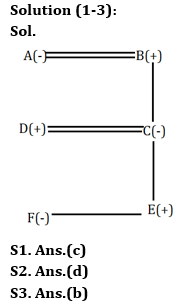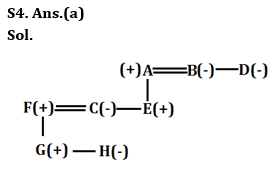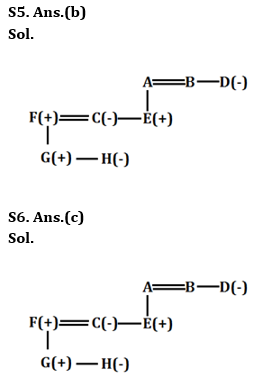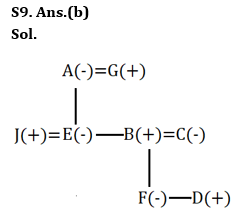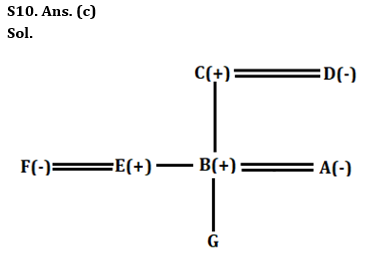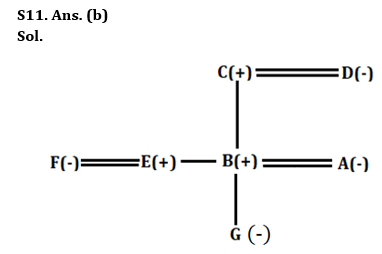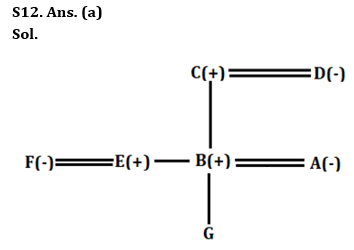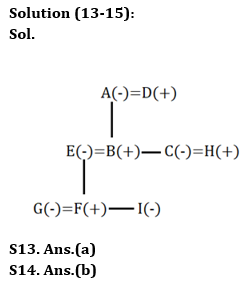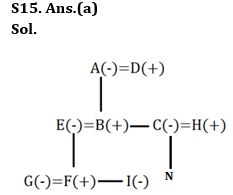## FAQs

### How many sections are there in the IRDAI exam?

There are 4 sections in the IRDAI assistant manager online preliminary exam. They are Reasoning, English Language, General awareness, Quantitative aptitude.

#### Congratulations!Union Budget 2023-24: Free PDF